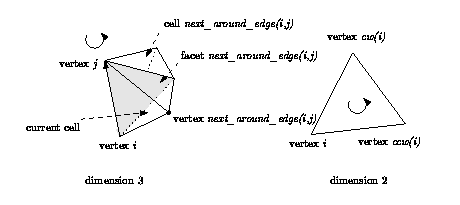## CGAL::Triangulation_utils_3

### Definition

The class Triangulation_utils_3 defines operations on the indices of vertices and neighbors within a cell.

#include <CGAL/Triangulation_utils_3.h>

### Operations

 unsigned int next_around_edge ( unsigned int i, unsigned int j) In dimension 3, index of the neighbor n that is next to the current cell, when turning positively around an oriented edge whose endpoints are indexed i and j. According to the usual numbering of vertices and neighbors in a given cell, it is also the index of the vertex opposite to this neighbor n. (see Figure). Precondition: ( i < 4 ) && ( j < 4 ) && ( i != j ). unsigned int ccw ( unsigned int i) Has a meaning only in dimension 2. Computes the index of the vertex that is next to the vertex numbered i in counterclockwise direction. (see Figure). Precondition: i<3. unsigned int cw ( unsigned int i) Same for clockwise.

Figure:  Operations on indices.